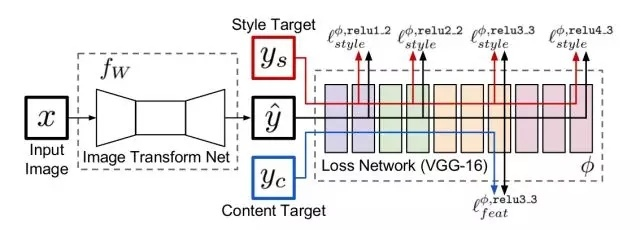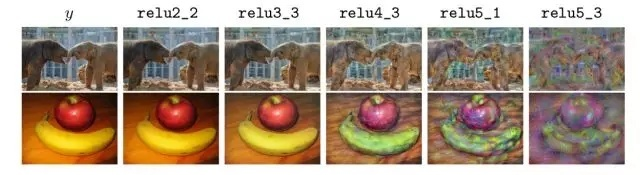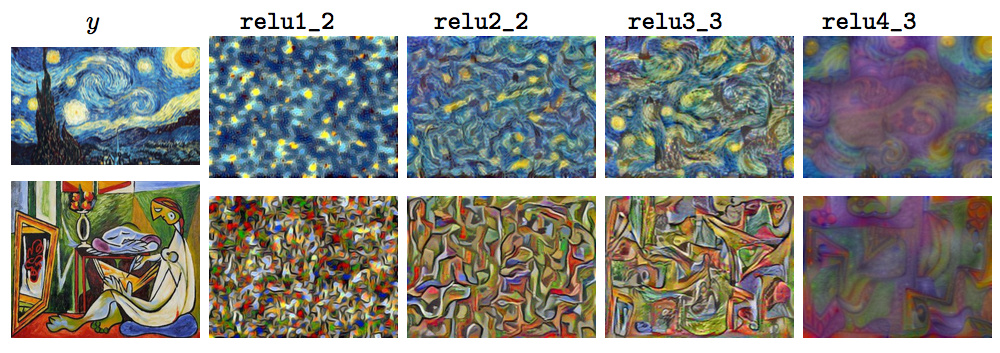2 构架3 网络细节

• 不使用pooling层，而是使用strided和fractionally strided卷积来做downsampling和upsampling，
• 使用了五个residual blocks
• 除了输出层之外的所有的非residual blocks后面都跟着spatial batch normalization和ReLU的非线性激活函数。
• 输出层使用一个scaled tanh来保证输出值在 $$[0, 255]$$ 内。
• 第一个和最后一个卷积层使用9×9的核，其他卷积层使用 $$3\times 3$$ 的核。

4 损失函数

4.1 Feature Reconstruction Loss

$$l^{\phi,j}_{feat}(\hat{y},y)={\frac {1}{C_jH_jW_j}}{\Vert {\phi_{j}(\hat{y})-\phi_{j}({y})} \Vert}^{2}_{2}$$

• $$j$$ 表示网络的第 $$j$$ 层
• $$C_jH_jW_j$$ 表示第 $$j$$ 层的 feature_map 的 size4.2 Style Reconstruction Loss

$$G^{\phi}_{j}(x)_{c,c′}={\frac {1}{C_jH_jW_j}}{\sum^{H_{j}}_{h=1}}{\sum^{W_{j}}_{w=1}}{\phi}_{j}(x)_{h,w,c}{\phi}_{j}(x)_{h,w,c′}$$

$$l^{\phi,j}_{style}(\hat{y},y)=\Vert {G_{j}^{\phi}(\hat{y})-G_{j}^{\phi}({y})} \Vert^{2}_{F}$$4.3 Simple Loss Function

1) Pixel Loss

pixel loss 是输出 $$\hat y$$ 和目标 $$y$$ 之间的欧几里得距离，只在网络有需要匹配的 ground-truth target $$y$$ 时才使用

$$l_{pixel}(\hat{y}, y)=\Vert {\hat{y}}-y \Vert^{2}_{2}$$

2）Total Variation Regularization

Total Variation Loss，实际上是一个平滑项（一个正则化项），目的是使生成的图像在局部上尽可能平滑，而它的定义和马尔科夫随机场（MRF）中使用的平滑项非常相似。

$$l_{TV}(\hat y)={\sum}_{n}{\Vert \hat{y}_{n+1}- \hat{y}_{n} \Vert}^{2}_{2}$$

• 其中 $$y_{n+1}$$ 是 $$y_n$$ 的相邻像素

5 基于Per-Pixel Loss的超分辨重建网络